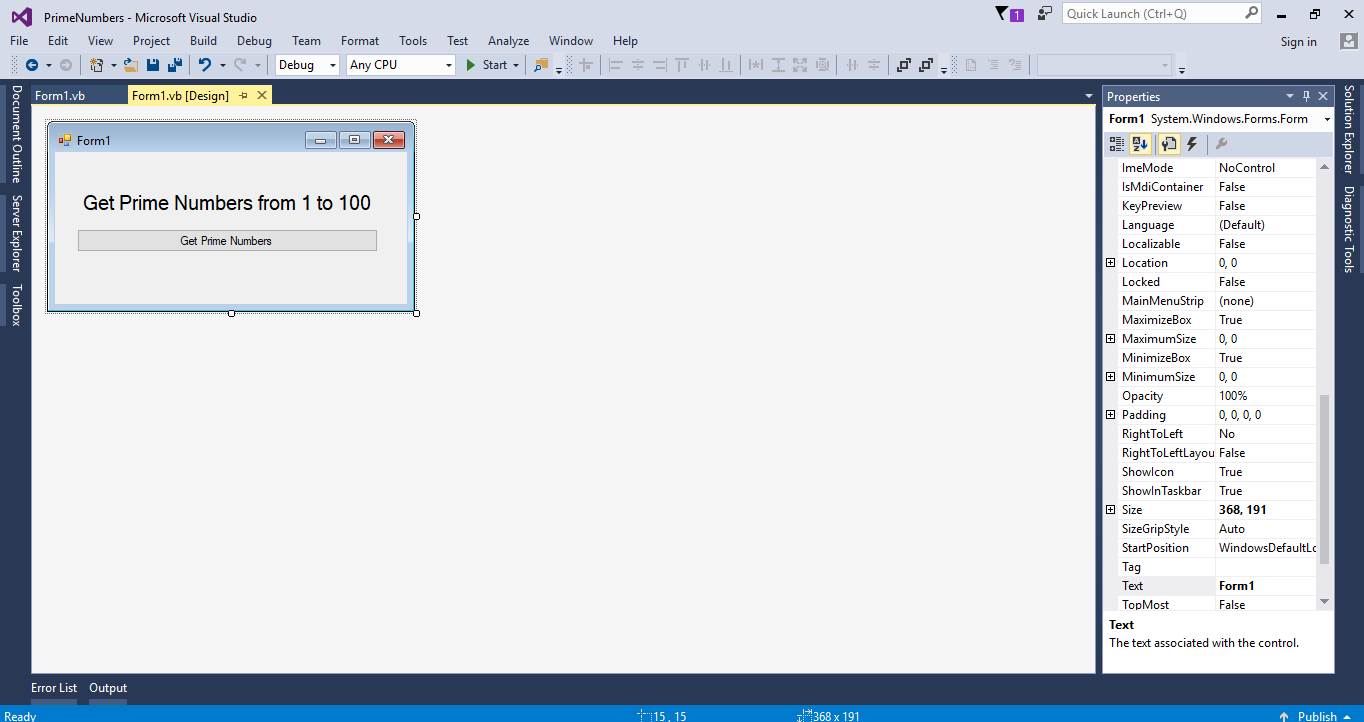# How to Get Prime Numbers in VB.Net

Body
Now, let’s create a simple program which is how to get prime numbers in VB.Net. You will learn here how to easily determine the prime numbers between 1 to 100. This simple program is also powerful that you can use to test large numbers to see if they are “prime”. Just follow the steps below.

## Creating Application

### Step 1

Open Microsoft Visual Studio 2015 and create a new windows form application. Then, add a Button and a Label in the Form just like shown below.### Step 2

Double click the Button to fire the `click event handler `of it and do the following codes for getting the prime numbers from 1 to 100.
`    Dim prime, numbers, i As Integer        prime = 1        MsgBox(“The prime Numbers are : ”)        For numbers = 1 To 100            For i = 2 To numbers - 1                If numbers Mod i = 0 Then                    prime = 0                    Exit For                Else                    prime = 1                End If             Next            If prime = 1 Then                MsgBox(numbers)            End If        Next`